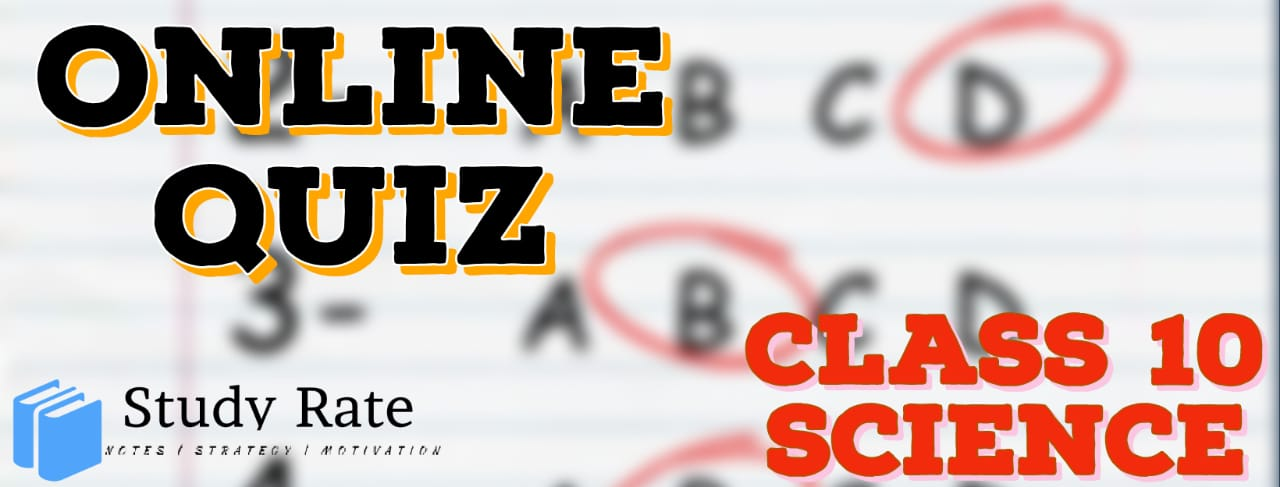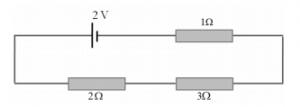## CBSE Class 10 Science Electricity MCQ Quiz with Answers

Free PDF Download of CBSE Class 10 Science Chapter 12 Electricity Multiple Choice Questions with Answers. MCQ Questions for Class 10 Science with Answers were Prepared Based on Latest Exam Pattern. Students can solve NCERT Class 10 Science Electricity Multiple Choice Questions with Answers to know their preparation level.

Electricity Class 10 Science MCQ Quiz Online

1. What is the amount of current flowing through an electric press, if the amount of charge passing through a conductor in 10 minutes is 300 C?

2. An electrical appliance has a resistance of 25 Ω. When this electrical appliance is connected to a 230 V supply line, the current passing through it will be:

3. When a 4 Ω resistor is connected across the terminals of a 2 V battery, the number of coulombs passing through the resistor per second is:

4. Keeping the potential difference constant, the resistance of the circuit is halved. The current will become:

5. If in the given arrangement, the three resistors are to be replaced by a single resistor. What will be the value of this resistor?6. The potential difference across the 3 Ω resistor is:7. A wire of resistance R1 is cut into five equal pieces. These five pieces of wire are then connected in parallel. If the resultant resistance of this combination be R2, then the ratio R1/R2 is:

8. Two appliances of rating 200 watt-250 volts and 100 watt-250 volts are joined in series to a 250 volts supply. Total power consumed in the circuit is

9. Two electric bulbs have resistances in the ratio 1:2. If they are joined in series, the energy consumed in them is in the ratio.

10. At a given time, a house is supplied with 100 A at 220 V. How many 75 W, 220 V light bulbs could be switched on in the house at the same time (if they are all connected in parallel)?